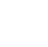Back to Chapter
Getting StartedBasic Syntax & DeclarationControl StatementsNo items found.
Function & EventsNo items found.
Array and its TypesNo items found.
OOP : Object Oriented ProgrammingNo items found.
Javascript StandardsNo items found.
HTML DOMNo items found.No items found.No items found.No items found.

# Break & Continue

## Break

Break Statement is used to Exit From the Loop immediately after encounter.

For Example :

You Created a Loop for sum of 100 natural numbers, but you want to exit the loop when the sum reaches to 100, then you have to use Break

## Continue

The continue statement is used to skip the current iteration of the loop.

For Example :

You Created a Loop for sum of 100 natural numbers, but you dont want to add 50 to the loop, then you have to use continue

# Syntax

## Break :

``` break: ```

### Example :

We will be doing example that we have discussed about i.e.

sum of 100 natural numbers until the sum reaches 1000

``` var sum = 0; var numbers = 100; var i=1; while(i <= numbers) { sum += i; if(sum>1000){ break; } i++; } console.log(sum); //output: //1035 ```

Explanation :

# Syntax

## Continue :

``` continue; ```

### Example :

We will be doing example that we have discussed about i.e.

sum of 100 natural numbers until the sum reaches 1000

``` var sum = 0; var numbers = 100; var i=0; while(i <= numbers) { i++; if(i==50){ continue; } sum += i; } console.log(sum); //output: //5000 ```

Explanation :